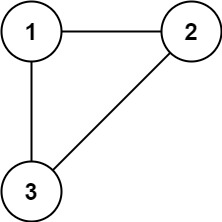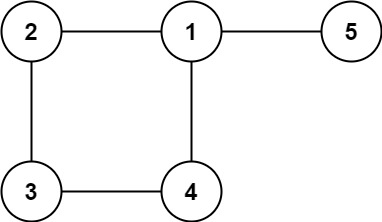## Algorithm

Problem Name: 684. Redundant Connection

In this problem, a tree is an undirected graph that is connected and has no cycles.

You are given a graph that started as a tree with `n` nodes labeled from `1` to `n`, with one additional edge added. The added edge has two different vertices chosen from `1` to `n`, and was not an edge that already existed. The graph is represented as an array `edges` of length `n` where `edges[i] = [ai, bi]` indicates that there is an edge between nodes `ai` and `bi` in the graph.

Return an edge that can be removed so that the resulting graph is a tree of `n` nodes. If there are multiple answers, return the answer that occurs last in the input.

Example 1:```Input: edges = [[1,2],[1,3],[2,3]]
Output: [2,3]
```

Example 2:```Input: edges = [[1,2],[2,3],[3,4],[1,4],[1,5]]
Output: [1,4]
```

Constraints:

• `n == edges.length`
• `3 <= n <= 1000`
• `edges[i].length == 2`
• `1 <= ai < bi <= edges.length`
• `ai != bi`
• There are no repeated edges.
• The given graph is connected.

## Code Examples

### #1 Code Example with C++ Programming

```Code - C++ Programming```

``````
class Solution {
public:
vector findRedundantConnection(vector>& edges) {
int tag = 1;
unordered_mapid;
unordered_map>g;
for(auto x: edges){
int u = x, v = x;
g[u].push_back(v);
g[v].push_back(u);
if(id[u] && id[v] && id[u] == id[v]) return x;
else if(!id[u] && !id[v]) id[u] = id[v] = tag++;
else if(id[u]) DFS(g, id, v, id[u]);
else id[u] = id[v];
}
}

void DFS(unordered_map>& g, unordered_map& id, int root, int tag){
if(id[root] == tag) return;
id[root] = tag;
for(auto x: g[root]) DFS(g, id, x, tag);
}
};
``````
Copy The Code &

Input

cmd
edges = [[1,2],[1,3],[2,3]]

Output

cmd
[2,3]

### #2 Code Example with Javascript Programming

```Code - Javascript Programming```

``````
const findRedundantConnection = function (edges) {
const uf = {}
for (let edge of edges) {
let u = edge
let v = edge
if (find(u) === find(v)) {
return edge
} else {
union(u, v)
}
}
function union(a, b) {
uf[find(a)] = uf[find(b)]
}
function find(x) {
if (!uf[x]) uf[x] = x
if (uf[x] === x) return x
return find(uf[x])
}
}
``````
Copy The Code &

Input

cmd
edges = [[1,2],[1,3],[2,3]]

Output

cmd
[2,3]

### #3 Code Example with Python Programming

```Code - Python Programming```

``````
class Solution:
def findRedundantConnection(self, edges: List[List[int]]) -> List[int]:
parent =  * len(edges)

def find(x):
if parent[x] == 0:
return x
parent[x] = find(parent[x])
return parent[x]

def union(x, y):
rootX = find(x)
rootY = find(y)
if rootX == rootY:
return False
parent[rootX] = rootY
return True

res = [0, 0]
for x, y in edges:
if not union(x - 1, y - 1):
res =  [x, y]
return res
``````
Copy The Code &

Input

cmd
edges = [[1,2],[2,3],[3,4],[1,4],[1,5]]

Output

cmd
[1,4]